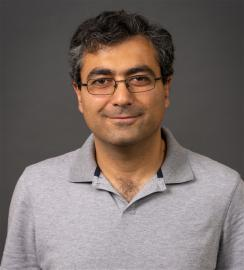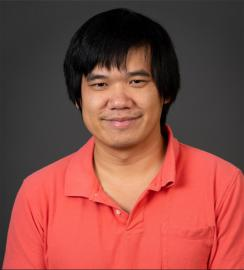Algebra is the study of structures with operations resembling addition and/or multiplication, but more general than the usual number system of real numbers. The most important of these structures are groups, rings, and fields.

The seeds of modern algebra can be found in the Abel-Ruffini Theorem that no general formula for the zeroes of a polynomial equation of degree 5 is possible. The modern proof uses Galois Theory, which studies the zeroes of a polynomial by associating a group of symmetries.

Because of its generality, algebra is used in many other fields of mathematics. Algebraic topology uses algebraic objects to study the properties of topological spaces.   Algebraic number theory studies various rings that generalize the set of integers.  Commutative ring theory underlies all of the structures of algebraic geometry.  The symmetries of smooth manifolds form objects called Lie groups.

The subject has many applications to the physical sciences, computer science, and engineering.  For example, one important application of finite fields is the theory of error-correcting codes, which is used to transmit information between devices without data loss.

### Faculty##### Alireza Salehi Golsefidy

Research Areas

Number Theory

Combinatorics

Arithmetic lattices

Homogeneous dynamical systems##### Daniel Rogalski

Research Areas

Noncommutative Ring Theory

Noncommutative Geometry##### Steven Sam

Research Areas

Algebraic Combinatorics